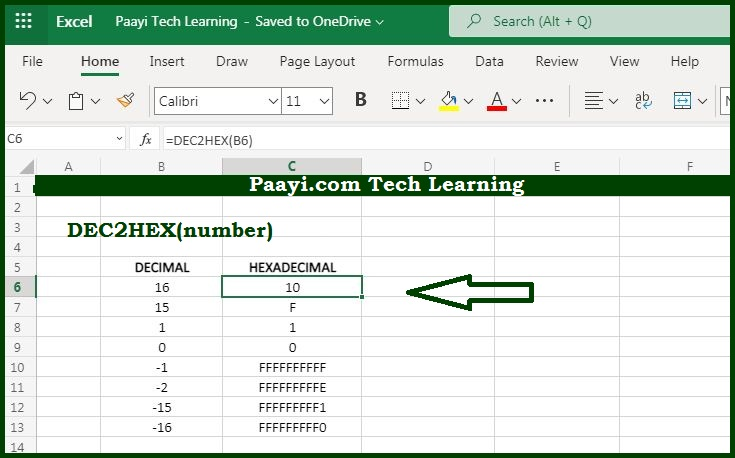# Learn How to Use Microsoft Excel DEC2HEX Function

Written by | 0 Comments | 674 Views

In this article, you will learn how to use the Microsoft Excel DEC2HEX function and its prime function in Microsoft Excel. You will also get to know the Microsoft Excel DEC2HEX function return value and syntax with the help of some examples.

Microsoft Excel DEC2HEX Function

The prime function of the Microsoft Excel DEC2HEX function is to convert decimal numbers to its hexadecimal equivalent. That means with the help of the DEC2HEX function you can able to convert a decimal number that represents its hexadecimal equivalent.

Return Value of DEC2HEX Function

The return value will be the hexadecimal number.

Syntax of DEC2HEX Function

=DEC2HEX(number, [places])

Where the arguments:

• number: It is the decimal number that you want to convert into hexadecimal equivalent.
• places: Holds the resulting hexadecimal number with zeros up to the specified number of digits. In case omitted returns the least number of characters required to represent the number (optional).

## How to Use Microsoft Excel DEC2HEX Function?As we know that DEC2HEX function used to convert the specified decimal number to its hexadecimal counterpart. Just make sure that the input must be the valid decimal number within the range of -2^39 to 2^39 - 1.